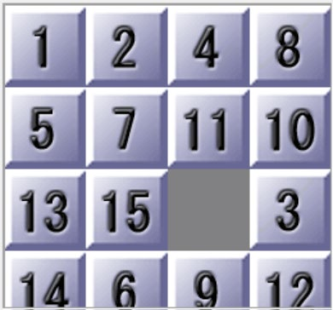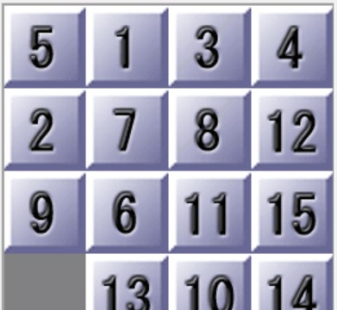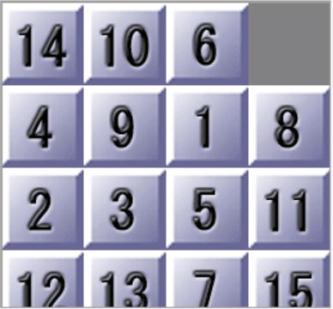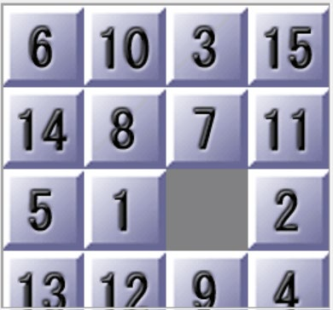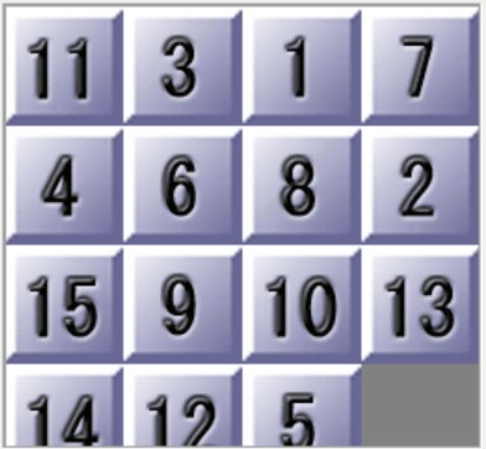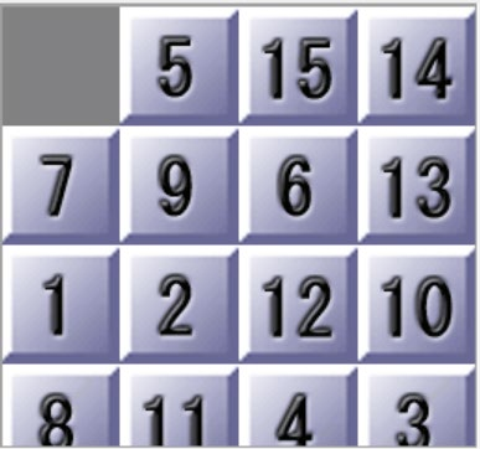# 【人工智能】盲目搜索与启发式搜索

## 如何形式化一个搜索问题

• 初始状态 initial state
• 行动 Actions
• 转移模型 transition model
• 目标测试 goal test
• 路径耗散 path cost

A solution to a problem is an action sequence that leads from the initial state to a goal state.

## 盲目搜索

• 宽度优先搜索
• 一致代价搜索
• 深度优先搜索
• 深度受限搜索
• 迭代加深搜索
• 双向搜索

• 算法流程图• 算法流程图• 算法伪代码

``````function Depth-Limited-Search(problem, limit)
if problem.Goal-Test(node.State) then return Solution(node)
if limit = 0 then return cutoff    //no solution
cutoff_occurred? <- false
for each action in problem.Action(node.State) do
child <- Child-Node(problem, node, action)
result <= Recusive-DLS(child, problem, limit - 1)
if result = cutoff then cutoff_occurred ? <- true
else if result ≠ failure then return result
if cutoff_occurred ? then return cutoff    //no solution
else return failure

fuction Iterative-Deepening-Search(problem, limit)
for depth = 0 to ∞ do
result <- Depth-Limited-Search(problem, limit)
if result ≠ cutoff then return result``````

## 启发式搜索

A*算法可以看作是BFS算法的升级版，在原有的BFS算法的基础上加入了启发式信息。
• 算法描述

• 算法优缺点

• 算法步骤

1.从起点开始，把其当成待处理点存入一个“开启列表”。
2.搜寻起点周围可能通过的节点，也把它们加入开启列表，为这些节点计算f(x)，g(x)h(x)，并且将节点A存为“父节点”。
3.从开启列表中删除节点A，将其加入“关闭列表”(列表中保存所有不需要再次检查的节点)。

4.从“开启列表”中找到估价函数值最低的节点C，并将它从“开启列表”中删除，添加到“关闭列表”中。
5.检查C所有相邻节点，将其加入“开启列表”，将C作为它们的父节点。
6.如果新的相邻节点已经在“开启列表”，则更新它们的g(x)值
• 算法流程图IDA算法是迭代加深深度优先算法（IDS）的扩展。因为它不需要去维护表，因此它的空间复杂度远远小于A。在搜索图为稀疏有向图的时候，它的性能会比A*更好。
• 算法描述

• 算法优点

• 算法步骤

1.从开始节点C，计算所有邻居节点的估价函数，选取估价函数最小的节点作为下一个访问节点。
2.对于某个节点，如果估价函数大于阈值，则返回当前节点的估值函数值。
3.对于某个节点，如果是目标节点，则返回状态"到达”。
• 算法伪代码

``````function h():  //启发式函数

function IDA_search(node, g, bound):  //node为当前节点，g为当前节点的路径消耗，bound为阈值
f = g + h(node)
if f > bound:
return f
if node == E:  //E为终点
return FOUND
min = r
for succ in successors(node) do:
t = search(succ, g + cost(node, succ), bound)
if t == FOUND:
return FOUND
if t < min:
min = t
return min

bound = h(A)
while(True):
t = IDA_search(root, 0, bound)
if t == FOUND:
return bound
if t = ∞:
return NOT_FOUND
bound := t``````

## 【编程任务一】盲目搜索算法实现

• 报告要求：报告中①需要有对实现的策略的原理解释，②需要策略的实验效果（四个方面的算法性能）的对比和分析，③以及自己对不同策略优缺点，适用场景的理解和认识。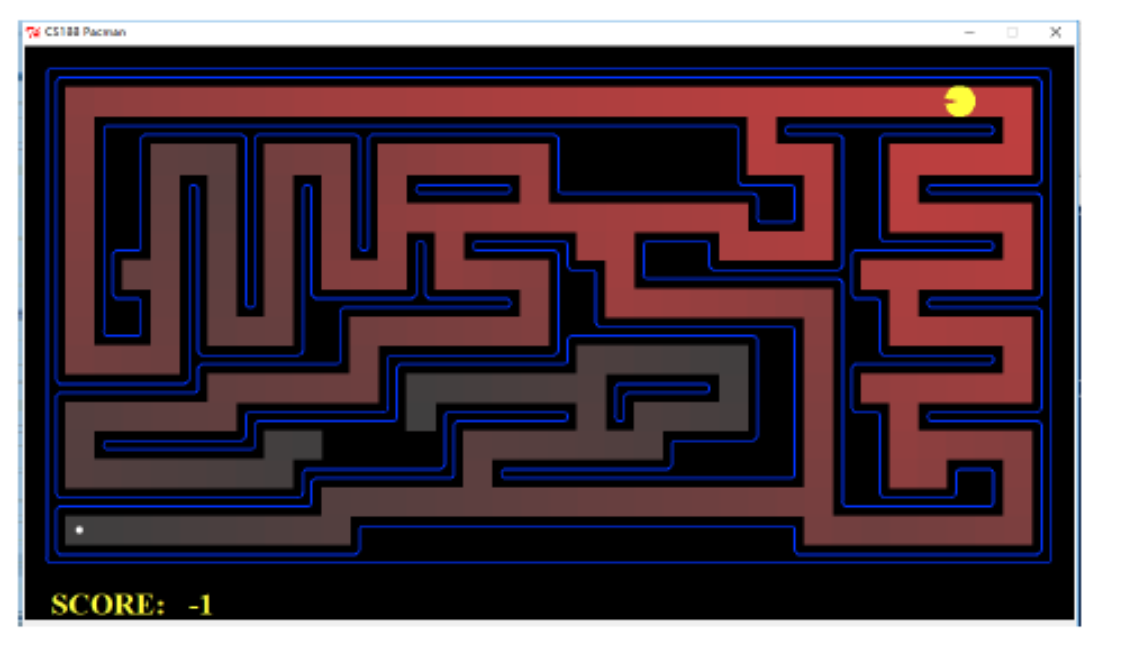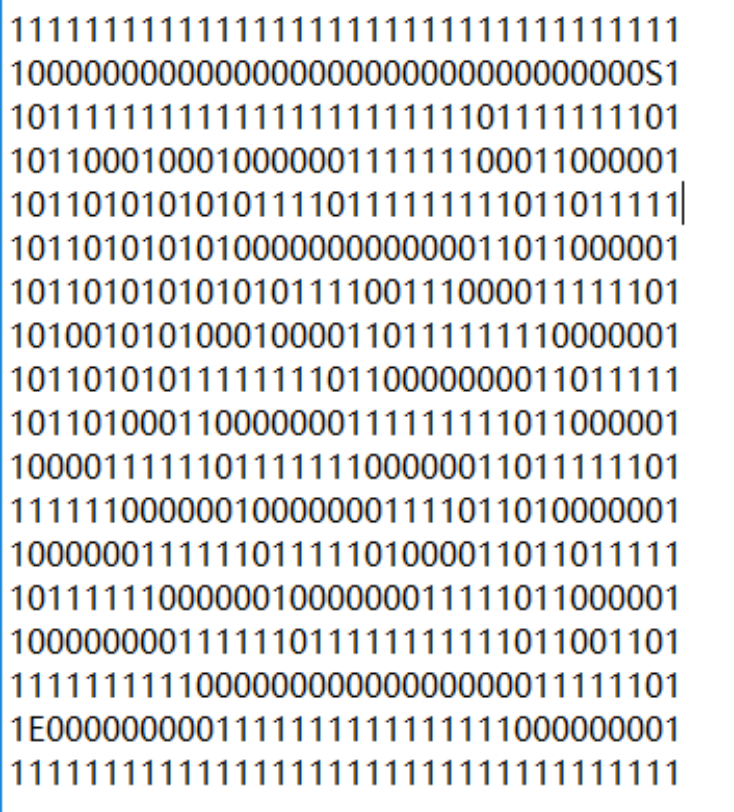S表示起点，E表示终点。1表示墙，0是可通行。

## 【编程任务二】启发式搜索算法实现

• 报告要求
①报告中需要包含对两个算法的原理解释
②需要包含性能和结果的对比和分析
③如果使用了多种启发式函数，最好进行对比和分析
④（可选）分情况分析估价值和真实值的差距会造成算法性能差距的原因